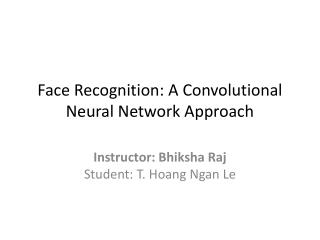# Face Recognition: A Convolutional Neural Network Approach - PowerPoint PPT PresentationDownload PresentationFace Recognition: A Convolutional Neural Network Approach

Face Recognition: A Convolutional Neural Network ApproachDownload Presentation## Face Recognition: A Convolutional Neural Network Approach

- - - - - - - - - - - - - - - - - - - - - - - - - - - E N D - - - - - - - - - - - - - - - - - - - - - - - - - - -
##### Presentation Transcript

1. Face Recognition: A Convolutional Neural Network Approach Instructor: BhikshaRajStudent: T. Hoang Ngan Le

2. The Problem Training Recognition Testing

3. Proposed System - Flowchart Identification Images • Dimensionality Reduction • Image Sampling • Convolutional Neural Network • Classification • Full Connected • Nearest Neighbor • Multi-layer Perceptron • SOM • KL transform

4. Image Sampling … A window is stepped over the image and a vector is created at each location.

5. Dimensionality Reduction - SOM 2 3 4 5 1 6

6. Dimensionality Reduction - KL Transform

7. Dimensionality Reduction - KL Transform • PCA • Objective function: • Karhunen-Loeve (KL) transform • Objective function:

8. Convolutional Network

9. Convolutional Network Motivation

10. Convolutional Network Convolution Subsample 1D local averaging operator 2D

11. Convolutional Network Layer 1 Layer 2

12. Convolutional Network Backpropagation gradient-descent procedure w13 w23 w33 w11 w12 w21 w22 w31 w32 Backpropagationalgorithm for standard MLP

13. Convolutional Neural Network - System Convolution Neural Network • K-L Transform • Image Sampling • Feature Extraction • MLP Style Classifier • Nearest – Neighbor Classifier • Classification • Multi-Layer Perceptron • SOM Dimensionality Reduction Images

14. Convolutional Neural Network – Extensions LeNet-5 http://yann.lecun.com/exdb/lenet/ C1,C3,C5 : Convolutional layer. 5 × 5 Convolution matrix. S2 , S4 : Subsampling layer. Subsampling by factor 2. F6 : Fully connected layer. About 187,000 connection. About 14,000 trainable weight

15. Convolutional Neural Network – Extension and variants Siamese CNNs Space Displacement Neural Networks (SDNN) Sparse Convolutional Neural Networks (Sparse CNN) Shunting Inhibitory ConvolutionalNeural Networks (SICoNNet)

16. Convolutional Neural Network – Experiment & Comparison 200 training images and 200 test images from ORL database (AT&T).

17. Comments • Convolutional Neural Networks are a special kind of multi-layer neural networks. • Like almost every other neural networks they are trained with a version of the back-propagation algorithm. • Convolutional Neural Networks are designed to recognize visual patterns directly from pixel images with minimal preprocessing. • Shared weights: all neurons in a feature share the same weights. • In this way all neurons detect the same feature at different positions. • Reduce the number of free parameters in the input image.